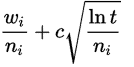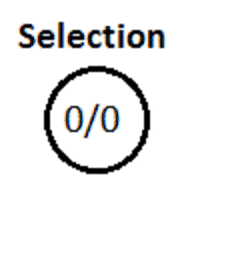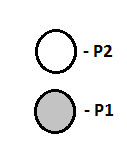### Get started with Spring and Spring Boot, through the Learn Spring course:

>> CHECK OUT THE COURSE

## 1. Overview

In this article, we’re going to explore the Monte Carlo Tree Search (MCTS) algorithm and its applications.

We’ll look at its phases in detail by implementing the game of Tic-Tac-Toe in Java. We’ll design a general solution which could be used in many other practical applications, with minimal changes.

## 2. Introduction

Simply put, Monte Carlo tree search is a probabilistic search algorithm. It’s a unique decision-making algorithm because of its efficiency in open-ended environments with an enormous amount of possibilities.

If you are already familiar with game theory algorithms like Minimax, it requires a function to evaluate the current state, and it has to compute many levels in the game tree to find the optimal move.

Unfortunately, it is not feasible to do so in a game like Go in which there is high branching factor (resulting in millions of possibilities as the height of the tree increases), and it’s difficult to write a good evaluation function to compute how good the current state is.

Monte Carlo tree search applies Monte Carlo method to the game tree search. As it is based on random sampling of game states, it does not need to brute force its way out of each possibility. Also, it does not necessarily require us to write an evaluation or good heuristic functions.

And, a quick side-note – it revolutionized the world of computer Go. Since March 2016, It has become a prevalent research topic as Google’s AlphaGo (built with MCTS and neural network) beat Lee Sedol (the world champion in Go).

## 3. Monte Carlo Tree Search Algorithm

Now, let’s explore how the algorithm works. Initially, we’ll build a lookahead tree (game tree) with a root node, and then we’ll keep expanding it with random rollouts. In the process, we’ll maintain visit count and win count for each node.

In the end, we are going to select the node with most promising statistics.

The algorithm consists of four phases; let’s explore all of them in detail.

### 3.1. Selection

In this initial phase, the algorithm starts with a root node and selects a child node such that it picks the node with maximum win rate. We also want to make sure that each node is given a fair chance.

The idea is to keep selecting optimal child nodes until we reach the leaf node of the tree. A good way to select such a child node is to use UCT (Upper Confidence Bound applied to trees) formula:In which

• wi = number of wins after the i-th move
• ni = number of simulations after the i-th move
• c = exploration parameter (theoretically equal to √2)
• t = total number of simulations for the parent node

The formula ensures that no state will be a victim of starvation and it also plays promising branches more often than their counterparts.

### 3.2. Expansion

When it can no longer apply UCT to find the successor node, it expands the game tree by appending all possible states from the leaf node.

### 3.3. Simulation

After Expansion, the algorithm picks a child node arbitrarily, and it simulates a randomized game from selected node until it reaches the resulting state of the game. If nodes are picked randomly or semi-randomly during the play out, it is called light play out. You can also opt for heavy play out by writing quality heuristics or evaluation functions.

### 3.4. Backpropagation

This is also known as an update phase. Once the algorithm reaches the end of the game, it evaluates the state to figure out which player has won. It traverses upwards to the root and increments visit score for all visited nodes. It also updates win score for each node if the player for that position has won the playout.

MCTS keeps repeating these four phases until some fixed number of iterations or some fixed amount of time.

In this approach, we estimate winning score for each node based on random moves. So higher the number of iterations, more reliable the estimate becomes. The algorithm estimates will be less accurate at the start of a search and keep improving after sufficient amount of time. Again it solely depends on the type of the problem.

## 4. Dry RunHere, nodes contain statistics as total visits/win score.

## 5. Implementation

Now, let’s implement a game of Tic-Tac-Toe – using Monte Carlo tree search algorithm.

We’ll design a generalized solution for MCTS which can be utilized for many other board games as well. We’ll have a look at most of the code in the article itself.

Although to make the explanation crisp, we may have to skip some minor details (not particularly related to MCTS), but you can always find the complete implementation on GitHub.

First of all, we need a basic implementation for Tree and Node classes to have a tree search functionality:

``````public class Node {
State state;
Node parent;
List<Node> childArray;
// setters and getters
}
public class Tree {
Node root;
}``````

As each node will have a particular state of the problem, let’s implement a State class as well:

``````public class State {
Board board;
int playerNo;
int visitCount;
double winScore;

// copy constructor, getters, and setters

public List<State> getAllPossibleStates() {
// constructs a list of all possible states from current state
}
public void randomPlay() {
/* get a list of all possible positions on the board and
play a random move */
}
}``````

Now, let’s implement MonteCarloTreeSearch class, which will be responsible for finding the next best move from the given game position:

``````public class MonteCarloTreeSearch {
static final int WIN_SCORE = 10;
int level;
int opponent;

public Board findNextMove(Board board, int playerNo) {
// define an end time which will act as a terminating condition

opponent = 3 - playerNo;
Tree tree = new Tree();
Node rootNode = tree.getRoot();
rootNode.getState().setBoard(board);
rootNode.getState().setPlayerNo(opponent);

while (System.currentTimeMillis() < end) {
Node promisingNode = selectPromisingNode(rootNode);
if (promisingNode.getState().getBoard().checkStatus()
== Board.IN_PROGRESS) {
expandNode(promisingNode);
}
Node nodeToExplore = promisingNode;
if (promisingNode.getChildArray().size() > 0) {
nodeToExplore = promisingNode.getRandomChildNode();
}
int playoutResult = simulateRandomPlayout(nodeToExplore);
backPropogation(nodeToExplore, playoutResult);
}

Node winnerNode = rootNode.getChildWithMaxScore();
tree.setRoot(winnerNode);
return winnerNode.getState().getBoard();
}
}``````

Here, we keep iterating over all of the four phases until the predefined time, and at the end, we get a tree with reliable statistics to make a smart decision.

Now, let’s implement methods for all the phases.

We will start with the selection phase which requires UCT implementation as well:

``````private Node selectPromisingNode(Node rootNode) {
Node node = rootNode;
while (node.getChildArray().size() != 0) {
node = UCT.findBestNodeWithUCT(node);
}
return node;
}``````
``````public class UCT {
public static double uctValue(
int totalVisit, double nodeWinScore, int nodeVisit) {
if (nodeVisit == 0) {
return Integer.MAX_VALUE;
}
return ((double) nodeWinScore / (double) nodeVisit)
+ 1.41 * Math.sqrt(Math.log(totalVisit) / (double) nodeVisit);
}

public static Node findBestNodeWithUCT(Node node) {
int parentVisit = node.getState().getVisitCount();
return Collections.max(
node.getChildArray(),
Comparator.comparing(c -> uctValue(parentVisit,
c.getState().getWinScore(), c.getState().getVisitCount())));
}
}``````

This phase recommends a leaf node which should be expanded further in the expansion phase:

``````private void expandNode(Node node) {
List<State> possibleStates = node.getState().getAllPossibleStates();
possibleStates.forEach(state -> {
Node newNode = new Node(state);
newNode.setParent(node);
newNode.getState().setPlayerNo(node.getState().getOpponent());
});
}``````

Next, we write code to pick a random node and simulate a random play out from it. Also, we will have an update function to propagate score and visit count starting from leaf to root:

``````private void backPropogation(Node nodeToExplore, int playerNo) {
Node tempNode = nodeToExplore;
while (tempNode != null) {
tempNode.getState().incrementVisit();
if (tempNode.getState().getPlayerNo() == playerNo) {
}
tempNode = tempNode.getParent();
}
}
private int simulateRandomPlayout(Node node) {
Node tempNode = new Node(node);
State tempState = tempNode.getState();
int boardStatus = tempState.getBoard().checkStatus();
if (boardStatus == opponent) {
tempNode.getParent().getState().setWinScore(Integer.MIN_VALUE);
return boardStatus;
}
while (boardStatus == Board.IN_PROGRESS) {
tempState.togglePlayer();
tempState.randomPlay();
boardStatus = tempState.getBoard().checkStatus();
}
return boardStatus;
}``````

Now we are done with the implementation of MCTS. All we need is a Tic-Tac-Toe particular Board class implementation. Notice that to play other games with our implementation; We just need to change Board class.

``````public class Board {
int[][] boardValues;
public static final int DEFAULT_BOARD_SIZE = 3;
public static final int IN_PROGRESS = -1;
public static final int DRAW = 0;
public static final int P1 = 1;
public static final int P2 = 2;

// getters and setters
public void performMove(int player, Position p) {
this.totalMoves++;
boardValues[p.getX()][p.getY()] = player;
}

public int checkStatus() {
/* Evaluate whether the game is won and return winner.
If it is draw return 0 else return -1 */
}

public List<Position> getEmptyPositions() {
int size = this.boardValues.length;
List<Position> emptyPositions = new ArrayList<>();
for (int i = 0; i < size; i++) {
for (int j = 0; j < size; j++) {
if (boardValues[i][j] == 0)
}
}
return emptyPositions;
}
}``````

We just implemented an AI which can not be beaten in Tic-Tac-Toe. Let’s write a unit case which demonstrates that AI vs. AI will always result in a draw:

``````@Test
void givenEmptyBoard_whenSimulateInterAIPlay_thenGameDraw() {
Board board = new Board();
int player = Board.P1;
int totalMoves = Board.DEFAULT_BOARD_SIZE * Board.DEFAULT_BOARD_SIZE;
for (int i = 0; i < totalMoves; i++) {
board = mcts.findNextMove(board, player);
if (board.checkStatus() != -1) {
break;
}
player = 3 - player;
}
int winStatus = board.checkStatus();

assertEquals(winStatus, Board.DRAW);
}``````

• It does not necessarily require any tactical knowledge about the game
• A general MCTS implementation can be reused for any number of games with little modification
• Focuses on nodes with higher chances of winning the game
• Suitable for problems with high branching factor as it does not waste computations on all possible branches
• Algorithm is very straightforward to implement
• Execution can be stopped at any given time, and it will still suggest the next best state computed so far

## 7. Drawback

If MCTS is used in its basic form without any improvements, it may fail to suggest reasonable moves. It may happen if nodes are not visited adequately which results in inaccurate estimates.

However, MCTS can be improved using some techniques. It involves domain specific as well as domain-independent techniques.

In domain specific techniques, simulation stage produces more realistic play outs rather than stochastic simulations. Though it requires knowledge of game specific techniques and rules.

## 8. Summary

At first glance, it’s difficult to trust that an algorithm relying on random choices can lead to smart AI. However, thoughtful implementation of MCTS can indeed provide us a solution which can be used in many games as well as in decision-making problems.

As always, the complete code for the algorithm can be found over on GitHub.

### Get started with Spring and Spring Boot, through the Learn Spring course:

>> CHECK OUT THE COURSE